# Mathematical puzzle with numbers

Sudoku is undoubtedly one of the most popular puzzles in recent times, which has also had a vertiginous development. Everyone relates it to mathematics because you have to put numbers in their boxes, although its relationship to this science is deeper.

Since the summer of 2005 became known internationally, Sudoku has become a mass phenomenon. We have Sudokus in newspapers, Sudoku magazines, Sudoku books, Sudokus on all existing electronic devices (mobiles, tablets, computers, etc.), Sudoku games in toy stores, computer programs to create Sudokus, serial collections related to Sudoku in tobacconists, children's sudoku puzzles and a huge number of variants of the original.

Although surely the people who are reading this entry in the Scientific Culture Notebook will already know perfectly what a sudoku puzzle is, we will start by remembering the rules of this math hobby. Normal Sudoku consists of a 9 x 9 cell grid, divided into 9 regions of 3 x 3 cells, and you have to fill in the 81 cells with the figures from 1 to 9 (starting from an initial situation in which some numbers are already placed in some of the cells), so that no figure can be repeated in the same row, column or region. Sudoku is related to Latin squares (see entry Latin squares, mathematics and abstract art or the book From chess to graphs, the mathematical seriousness of games ) studied by the mathematician Leonard Euler (1707-1783), although the modern game was created in the 1970s by retired architect and hobby designer Howard Garns (1905-1989) and published under the name number place in the magazine Dell Pencil Puzzles & Word Games .

Maki Kaji, president of the Nikoli publishing house, specialized in games and hobbies, in particular logic puzzles, exported it to Japan and began publishing it in 1984 in his magazine Monthly Nikolist under the name Suji wa dokushin ni kagiru (numbers must be alone), which was shortened to Su Doku . Its expansion to the rest of the world came from the hand of the retired New Zealand judge, resident in Hong Kong, Wayne Gould, who developed a computer program to quickly create sudokus. In 2004, sudokus began to be published in British newspapers, such as The Times and The Guardian and ended up becoming, since 2005, a very popular puzzle that appeared in most of the newspapers of the world.

The relationship between Sudoku and mathematics is not that numbers are used, since letters, colors or any other symbols could be used, but rather that it is combinatorial, it is based on the relationship between the different positions of symbols (numbers) in rows, columns, and regions. If we look at the solution of a sudoku puzzle, like the previous image, it is a particular type of Latin square (remember that a Latin square of order n is a square lattice of size n x n in which each entry is a number from 1 to n such that each number of appears once , and only once, in each row and each column) of size 9 x 9, in which it is also verified that the numbers from 1 to 9 appear only once in each of the nine 3 x 3 regions.

There are different mathematical questions involved in this puzzle, such as how many solution structures, that is, Latin squares of order 9 that satisfy the rule of different regions, are there (which turn out to be 6,670,903,752,021,072,936,960, although if we take into account the symmetries, these are reduced to 5,472,730,538 different sudoku solutions); given a Latin square of order 9 that is a sudoku solution, how many different sudoku puzzles can be generated from it (that is, eliminating the numbers from the boxes until leaving a small initial amount that is the starting point of the game, the clues ) and what is the minimum amount of initial numbers (clues) that are needed for the sudoku to be well defined, that is, that there is a unique solution (which turn out to be 17 clues), among others.

However, my The intention in this entry in the Matemoción section of the Scientific Culture Notebook is not to talk about the mathematics of sudoku (see, for example, the article Sudoku and modeling by María Merino), but rather to present other puzzles Mathematics similar to this, in the sense that numbers are placed on a lattice structure.

In 2010, Jai Gomer of Kobayaashi Studios developed a series of number puzzles, called sujiko and suko, h erederos of the sudoku, but they already involve some arithmetic – specifically the addition – in their rules. These first appeared in English newspapers such as The Times and The Telegraph and later in newspapers around the world, such as, El País .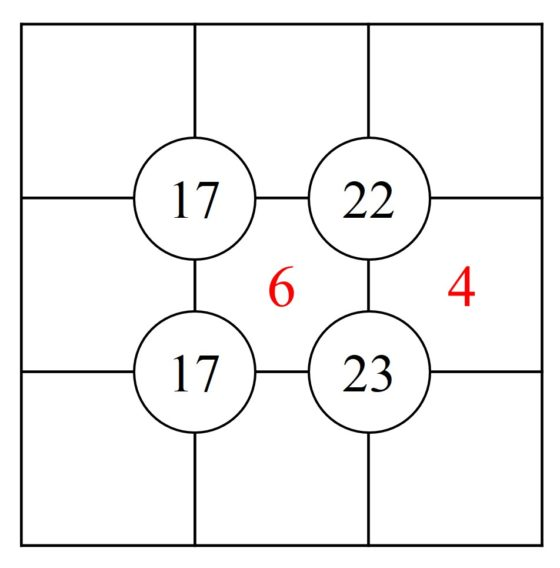The sujiko board is a 3 x 3 grid, with four circular spaces placed at the four intersections of the horizontal and vertical lines of the grid, on which four numbers are written (for example, 17, 22, 17, 23, in the previous image). The objective of the game is to place the numbers from 1 to 9 in the cells – although there may already be some placed, as a clue (in the previous sujiko 6 and 4) – so that the sum of the numbers that are in the boxes around each circle is exactly the number written on it.

This puzzle is solved logically, like Sudoku, but taking into account its rule, that the sum of the numbers of the cells around a circle is the value of the circle. Let's see how to solve the previous sujiko, which is one of the simple ones.

The numbers in the two boxes on the top right must add up to 12, since 6 and 4 are also around 22 and their sum is 6 + 4 = 10. As in those boxes there cannot be 6 and 4, which they are already placed, the two options are 9 and 3, or 7 and 5, without determining yet which one goes in each of the two boxes. If we carry out the same reasoning for the two cells at the bottom right, which must add up to 13, the only possibility is 8 and 5. As the number 5 would be here, in the two cells above it would have to be the numbers 9 and 3.

Let's see the order above. In the central box of the row above there is 3 or 9, if it were 9 we would have that around the box of 17 it would add 9 + 6 = 15, then the other two boxes should add 2, which is impossible , since the lowest possible sum would be 1 + 2 = 3. In conclusion, in the first row, the number 3 would go in the central box and 9 in the right.

Before continuing, let's think about three numbers We are missing to use for the cells in the left column. It would be 1, 2 and 7. So, around the top 17 we have 3 + 6 = 9, plus the sum of the numbers in the two cells, which should be 8, then the numbers in those two cells are 1 and 7. Yes We follow this reasoning a little more, we will get the definitive solution, which appears in the image below.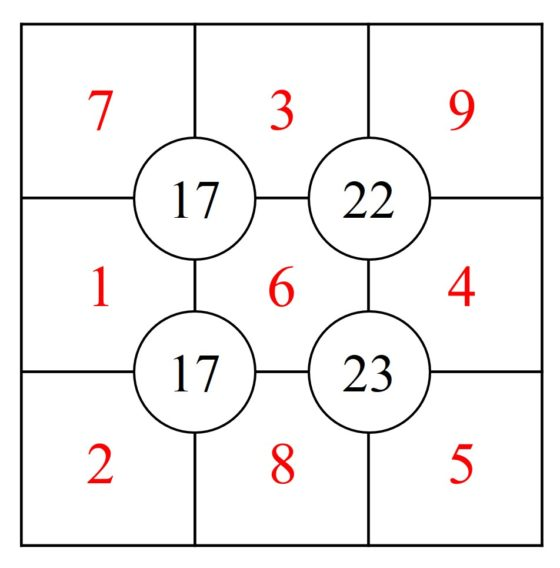We can present this problem in the following way. Its information is given in the following diagram, where in the cells we have the game variables and in the circles the game data.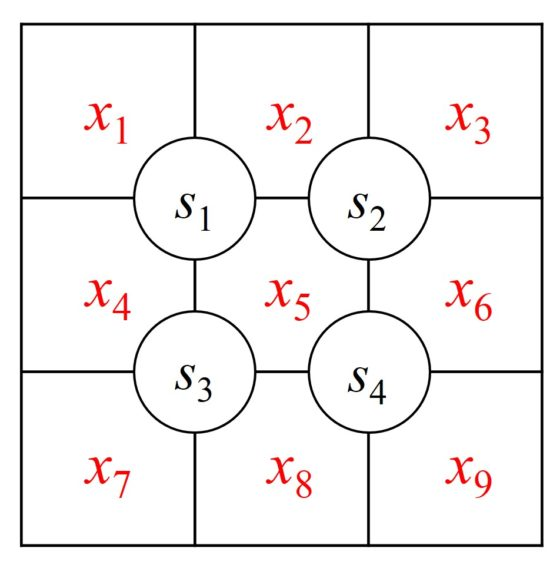Then the sujiko solution is the solution of a system of four equations and nine unknowns, although with the following restrictions, the variables only take values ​​between the numbers 1, 2, 3, 4, 5 , 6, 7, 8, 9 and each variable takes a different value from the others.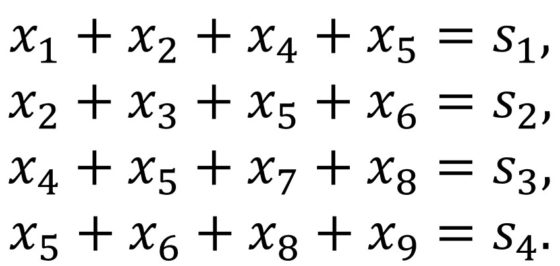Although the fun of the sujiko is to obtain the solution logically, the algebraic approach can also be used as Example for students who are working on linear algebra for solving systems of linear equations.

I leave you with two sujikos, of medium and high levels, that I have taken from the page Sudokasana where they call Minisum puzzles . Although you can also find sujikos in the newspaper's hobby page The Times .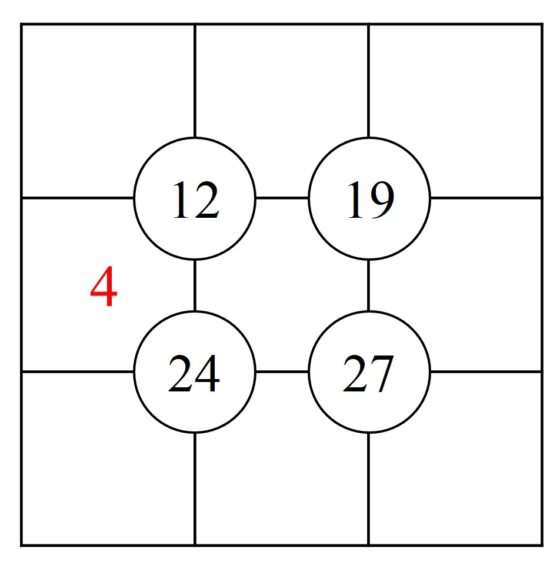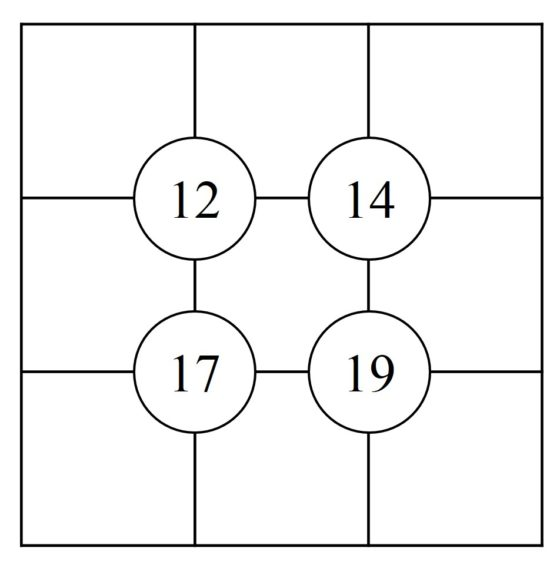On the other hand, the suko puzzle is like the sujiko, but at the same time that a new condition related to the 3 x 3 grid regions is added. The condition is that the sum of the numbers of the cells of the same color add up to the amount indicated in the game, as it appears in the following image (suko that we have taken from the hobby page of The Times ).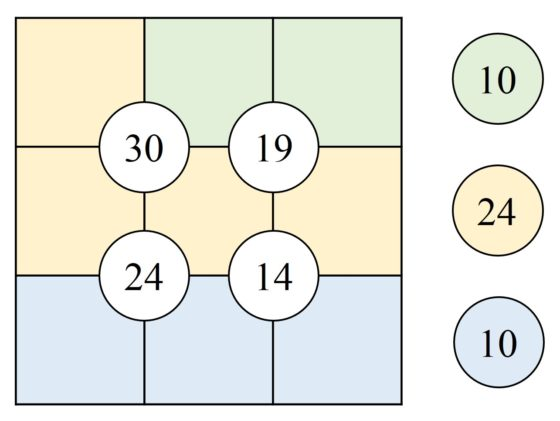From the algebraic point of view, in suko we add three more linear equations, then we have a system of seven linear equations with nine unknowns, and the solution to the puzzle is the solution of the system of equations.

Another puzzle related to sudoku, or rather Latin squares, but that adds arithmetic – although now the four arithmetic operations, not just the sum- to its rules is the KenKen.

The KenKen puzzle, also known by the names KenDoku, MathDoku or CalcuDoku, was invented by the Japanese mathematics teacher Tetsuya Miyamoto as a tool to exercise the brain. Its name is derived from the Japanese word Ken which means intelligence or ingenuity.

In 2007 the toy inventor Robert Fuhrer, owner of the toy company Nextoy discovered several books with this hobby and his interest in it would cause the logic puzzle to end up in the pages of the British newspaper The Times and later in many other newspapers around the world.

The KenKen rules are as follows. following. We start from a grid n x n on which we must place the numbers from 1 to n so that in each row and each column are all the n numbers and none are repeated (then it will be a Latin square of order n ), but in addition the grid is divided into a series of regions, in each of which appear a arithmetic operation (addition, subtraction, multiplication or division) and a number, which will be the result of applying the indicated arithmetic operation to the numbers of the cells in that region.

In the following example of a KenKen puzzle we have a 4 x 4 grid, then we have to write the numbers 1, 2, 3 and 4 in the cells of the same, so that a Latin square is constituted – in each row and each column appears each of the four numbers once and only once – and the arithmetic conditions of the regions are met – for example, in the region at the top left the division of the two numbers is 2, or in the region of on the right the subtraction of the two numbers is also 2–.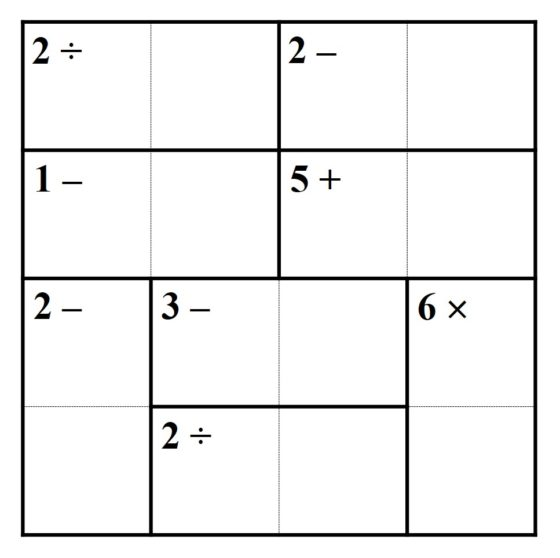The solution for this KenKen is given in the following image.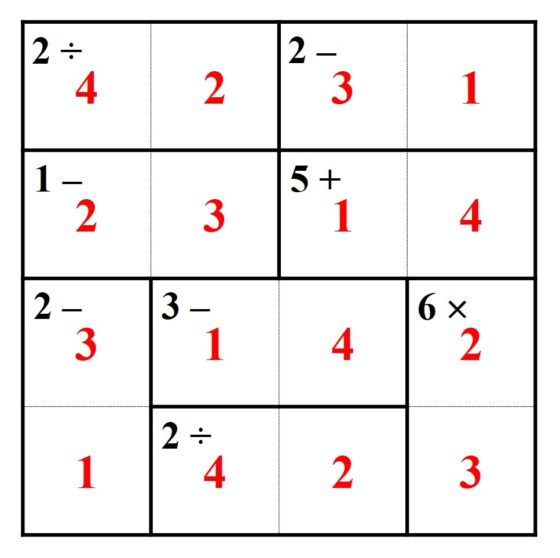On the page KenKen, puzzles that make you smarter you can find puzzles with grids from 3 x 3 to 9 x 9, of different levels of difficulty. We leave you with one for you to have fun of size 6 x 6 and medium difficulty.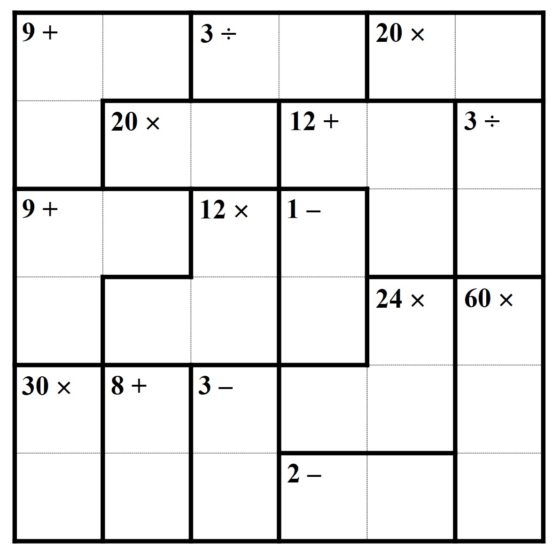Another of the games from Nikoli publishing house, internationally famous for Sudoku, is Hitori, a term that means in Japanese "lonely". This hobby consists of a grid with numbers in all its cells and its action consists of eliminating a series of numbers, or painting the corresponding cells black, so that the following rules are met:

i) in each row and each column does not repeat any number;

ii) the crossed out or black cells cannot be adjacent (they can touch corner to corner, but not side to side);

iii) the rest of the cells with numbers have They must be connected, vertically or horizontally, to each other, that is, isolated cells cannot remain.

Let's see what the game consists of using a concrete example of size 5 x 5.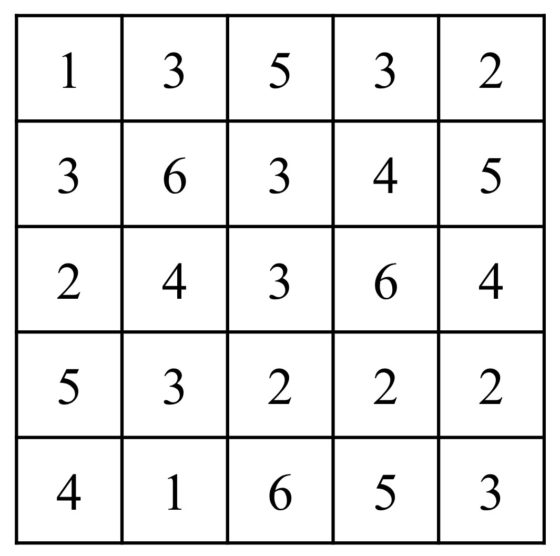The solution appears in the following image. As we can see, all three conditions of this logic puzzle are met. In each row and each column of the solution no number is repeated, for example, in the first row there were two cells with the number 3, then one had to be crossed out. Striped cells are only touched at the vertices or not touched. And there are no cells isolated from the others.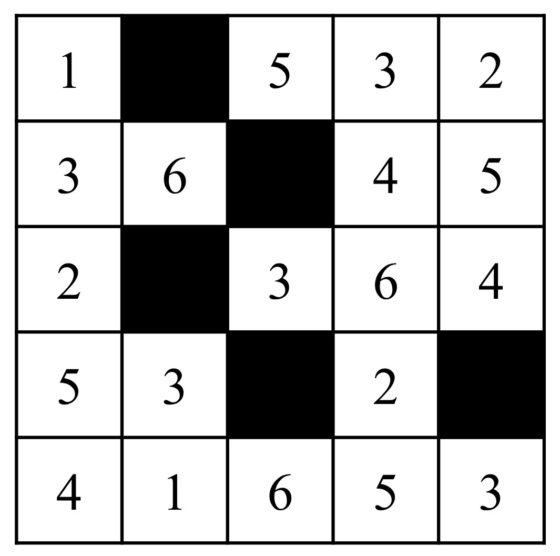On page Hitori Conquest [www.hitoriconquest.com/] you can find more Hitori puzzles of sizes 5 x 5, 8 x 8 and 12 x 12, like the one we leave below for those who want to have a good time solving it.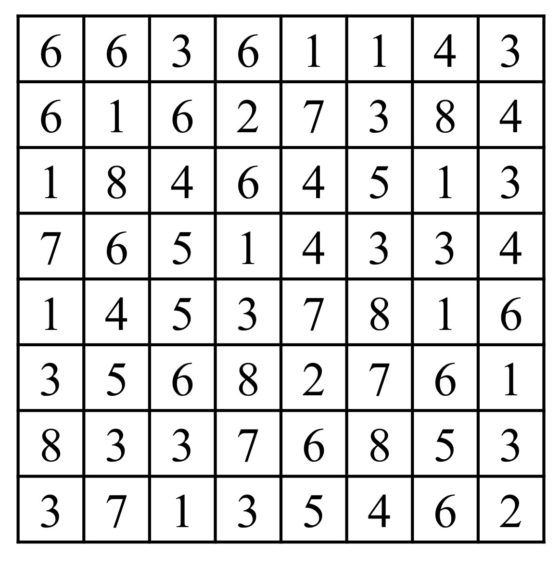We are going to conclude this entry in the Scientific Culture Notebook with another of the logic puzzles popularized by the Japanese publishing house Nikoli known as Numberlink (which we could translate as “connects the numbers"). This game has its origin in classical recreational mathematics, since a version of it was proposed by the American recreational mathematician Sam Loyd (1841-1911) in 1897 and also by the English recreational mathematician Henry Dudeney (1857-1930), in his book Amusements in Mathematics (1917), problem 252, which we see in the following image.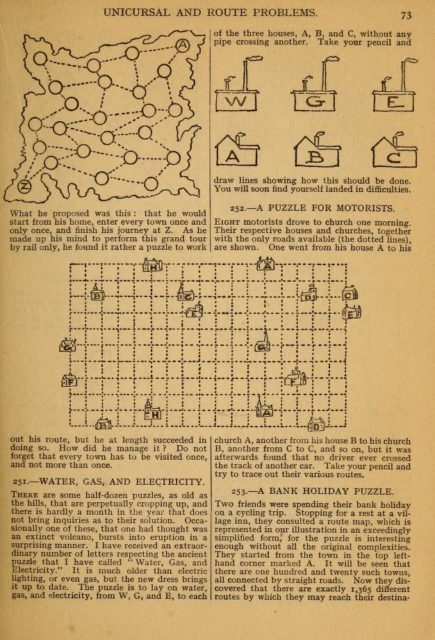The puzzle consists of a grid in which pairs of numbers appear (although also they could be letters, colors, or other symbols), two ones, two twos, two threes, and so on. The player has to connect each number with its equal by means of a line that passes from one cell to another, horizontally or vertically, in such a way that the lines cannot cross each other, nor go back to cells already traveled and should not no cell is left without being traversed by any line (although there are some game designers who skip this rule).

Let's see a simple example and its solution.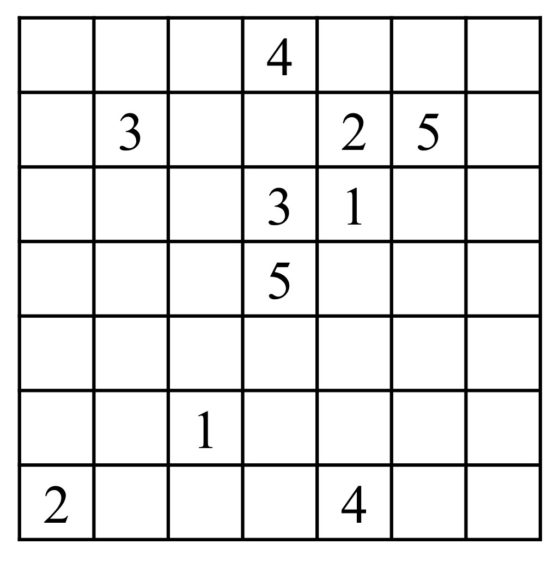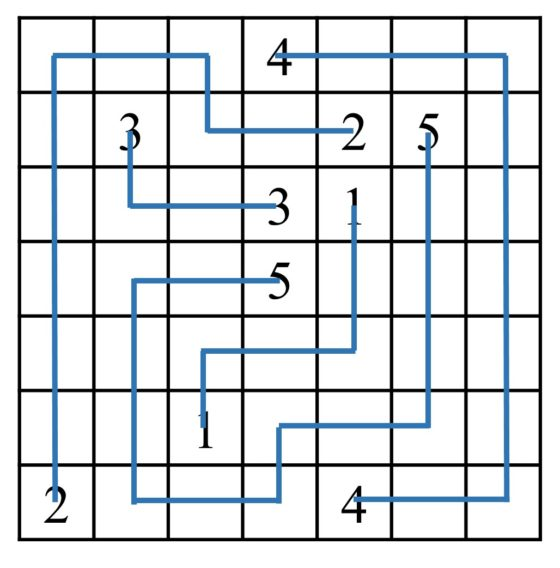We propose a logical puzzle to connect the numbers, taken from the page Puzzle's Baron Numberlinks for your enjoyment.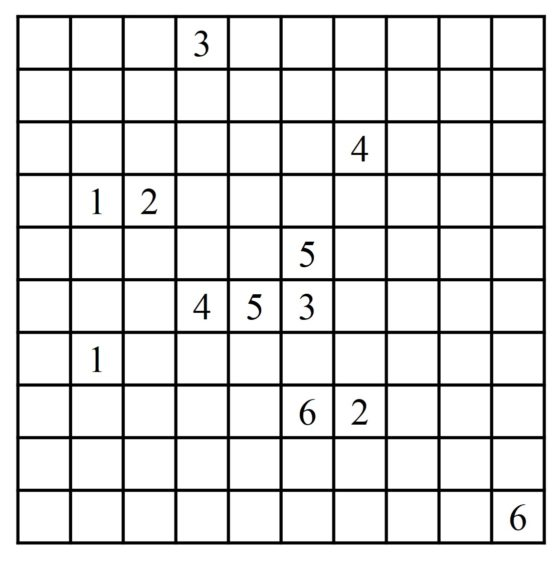For all the logic puzzles presented in this entry there are mobile applications that you can download and play at any time. While I was writing this entry I have downloaded some to my mobile to be able to play quietly.

Bibliography

1.- Raúl Ibáñez, Sudoku Mathematics in advertising, DivulgaMAT, 2011.

2.- María Merino, Sudokus and modeling A walk through Geometry 2009/2010, UPV / EHU, 2010.

3.- Raúl Ibáñez, From chess to graphs, the mathematical seriousness of games The world is mathematical collection, RBA, 2015.

About the author: Raúl Ibáñez is a professor at the Department of Mathematics at the UPV / EHU and collaborator of the Chair of Scientific Culture

The article Mathematical puzzles with numbers has been written in Notebook of Scientific Culture .Share